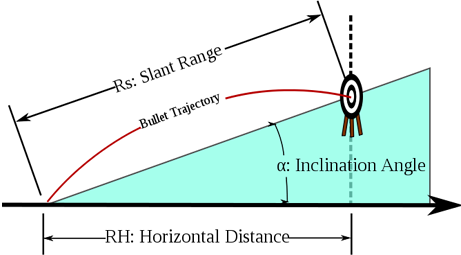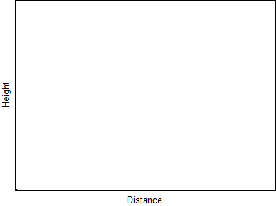TrajectoryBy Wikipedia,
the free encyclopedia,

http://en.wikipedia.org/wiki/Trajectory

A trajectory is the path a moving object follows through space. The object might be a projectile or a satellite, for example. It thus includes the meaning of orbit - the path of a planet, an asteroid or a comet as it travels around a central mass. A trajectory can be described mathematically either by the geometry of the path, or as the position of the object over time.

In control theory a trajectory is a time-ordered set of states of a dynamical system (see e.g. Poincaré map). In discrete mathematics, a trajectory is a sequence$(f^k(x))_{k \in \mathbb{N}}$ of values calculated by the iterated application of a mapping f to an element x of its source.Illustration showing the trajectory of a bullet fired at an uphill target.

## Physics of trajectories

A familiar example of a trajectory is the path of a projectile such as a thrown ball or rock. In a greatly simplified model the object moves only under the influence of a uniform homogenous gravitational force field. This can be a good approximation for a rock that is thrown for short distances for example, at the surface of the moon. In this simple approximation the trajectory takes the shape of a parabola. Generally, when determining trajectories it may be necessary to account for nonuniform gravitational forces, air resistance (drag and aerodynamics). This is the focus of the discipline of ballistics.

One of the remarkable achievements of Newtonian mechanics was the derivation of the laws of Kepler, in the case of the gravitational field of a single point mass (representing the Sun). The trajectory is a conic section, like an ellipse or a parabola. This agrees with the observed orbits of planets and comets, to a reasonably good approximation. Although if a comet passes close to the Sun, then it is also influenced by other forces, such as the solar wind and radiation pressure, which modify the orbit, and cause the comet to eject material into space.

Newton's theory later developed into the branch of theoretical physics known as classical mechanics. It employs the mathematics of differential calculus (which was, in fact, also initiated by Newton, in his youth). Over the centuries, countless scientists contributed to the development of these two disciplines. Classical mechanics became a most prominent demonstration of the power of rational thought, i.e. reason, in science as well as technology. It helps to understand and predict an enormous range of phenomena. Trajectories are but one example.

Consider a particle of mass m, moving in a potential field V. Physically speaking, mass represents inertia, and the field V represents external forces, of a particular kind known as "conservative". That is, given V at every relevant position, there is a way to infer the associated force that would act at that position, say from gravity. Not all forces can be expressed in this way, however.

The motion of the particle is described by the second-order differential equation$m \frac{\mathrm{d}^2 \vec{x}(t)}{\mathrm{d}t^2} = -\nabla V(\vec{x}(t))$ with$\vec{x} = (x, y, z)$

On the right-hand side, the force is given in terms of$\nabla V$, the gradient of the potential, taken at positions along the trajectory. This is the mathematical form of Newton's second law of motion: mass times acceleration equals force, for such situations.

## Examples

### Uniform gravity, no drag or windTrajectories of two objects thrown at the same angle. The blue object doesn't experience any drag and moves along a parabola. The black object experiences Stokes' drag.

The ideal case of motion of a projectile in a uniform gravitational field, in the absence of other forces(such as air drag), was first investigated by Galileo Galilei. To neglect the action of the atmosphere, in shaping a trajectory, would have been considered a futile hypothesis by practical minded investigators, all through the Middle Ages in Europe. Nevertheless, by anticipating the existence of the vacuum, later to be demonstrated on Earth by his collaborator Evangelista Torricelli, Galileo was able to initiate the future science of mechanics. And in a near vacuum, as it turns out for instance on the Moon, his simplified parabolic trajectory proves essentially correct.

In the analysis that follows we derive the equation of motion of a projectile as measured from an inertial frame, at rest with respect to the ground, to which frame is associated a right-hand co-ordinate system - the origin of which coincides with the point of launch of the projectile. The x-axis is parallel to the ground and the y axis perpendicular to it ( parallel to the gravitational field lines ). Let g be the acceleration of gravity. Relative to the flat terrain, let the initial horizontal speed be vh = vcos(θ) and the initial vertical speed be vv = vsin(θ). It will also be shown that, the range is 2vhvv / g, and the maximum altitude is$v_v^2/2g$; The maximum range, for a given initial speed v, is obtained when vh = vv, i.e. the initial angle is 45 degrees. This range is v / g, and the maximum altitude at the maximum range is a quarter of that.

#### Derivation of the equation of motion

Assume the motion of the projective is being measured from a Free fall frame which happens to be at (x,y)=(0,0) at t=0. The equation of motion of the projectile in this frame ( by the principle of equivalence) would be y = xtan(θ). The co-ordinates of this free-fall frame, with respect to our inertial frame would be y = − gt / 2. That is, y = − g(x / vh) / 2.

Now translating back to the inertial frame the co-ordinates of the projectile becomes y = xtan(θ) − g(x / vh) / 2 That is:$y=-{g\sec^2\theta\over 2v_0^2}x^2+x\tan\theta$,

(where v0 is the initial speed, h is the height the projectile is fired from, and g is the acceleration due to gravity).

#### Range and height

The range R of the projectile is found when the y-component of the above equation is zero.$0 = v_v t - g t^2/2 = t \left( v_v - g t/2\right)\,$

The equation gives solutions at t = 0 and t = 2vv / g (the hang-time of the projectile). The range is then R = 2vhvv / g.

From the symmetry of the parabola the maximum height occurs at the halfway point x = vhvv / g. Substituting in the above equation and solving for y, we have$v_v^2/2g$.

#### Angle of elevation

In terms of angle of elevation θ and initial speed v:$v_h=v \cos \theta,\quad v_v=v \sin \theta \;$

giving the range as$R= 2 v^2 \cos(\theta) \sin(\theta) / g = v^2 \sin(2\theta) / g\,.$

This equation can be rearranged to find the angle for a required range${ \theta } =\frac 1 2 \sin^{-1} \left( { {g R} \over { v^2 } } \right)$ (Equation II: angle of projectile launch)

Note that the sine function is such that there are two solutions for θ for a given range dh. Physically, this corresponds to a direct shot versus a mortar shot up and over obstacles to the target. The angle θ giving the maximum range can be found by considering the derivative or R with respect to θ and setting it to zero.${\mathrm{d}R\over \mathrm{d}\theta}={2v^2\over g} \cos(2\theta)=0$

which has a non trivial solutions at$2\theta=\pi/2=90^\circ$. The maximum range is then$R_{max} = v^2/g\,$. At this angle sin(π / 2) = 1 so the maximum height obtained is${v^2 \over 4g}$.

To find the angle giving the maximum height for a given speed calculate the derivative of the maximum height H = vsin(θ) / (2g) with respect to θ, that is${\mathrm{d}H\over \mathrm{d}\theta}=v^2 \cos(\theta) /(2g)$ which is zero when$\theta=\pi=180^\circ$. So the maximum height$H_{max}={v^2\over 2g}$ is obtained when the projectile is fired straight up.

### Uphill/downhill in uniform gravity in a vacuum

Given a hill angle α and launch angle θ as before, it can be shown that the range along the hill Rs forms a ratio with the original range R along the imaginary horizontal, such that:$\frac{R_s} {R}=(1-\cot \theta \tan \alpha)\sec \alpha$ (Equation 11)

In this equation, downhill occurs when α is between 0 and -90 degrees. For this range of α we know: tan( − α) = − tanα and sec( − α) = secα. Thus for this range of α, Rs / R = (1 + tanθtanα)secα. Thus Rs / R is a positive value meaning the range downhill is always further than along level terrain. The lower level of terrain causes the projectile to remain in the air longer, allowing it to travel further horizontally before hitting the ground.

While the same equation applies to projectiles fired uphill, the interpretation is more complex as sometimes the uphill range may be shorter or longer than the equivalent range along level terrain. Equation 11 may be set to Rs / R = 1 (i.e. the slant range is equal to the level terrain range) and solving for the "critical angle" θcr:$1=(1-\tan \theta \tan \alpha)\sec \alpha \quad \;$$\theta_{cr}=\arctan((1-\csc \alpha)\cot \alpha) \quad \;$

Equation 11 may also be used to develop the "rifleman's rule" for small values of α and θ (i.e. close to horizontal firing, which is the case for many firearm situations). For small values, both tanα and tanθ have a small value and thus when multiplied together (as in equation 11), the result is almost zero. Thus equation 11 may be approximated as:$\frac{R_s} {R}=(1-0)\sec \alpha$

And solving for level terrain range, R$R=R_s \cos \alpha \$ "Rifleman's rule"

Thus if the shooter attempts to hit the level distance R, s/he will actually hit the slant target. "In other words, pretend that the inclined target is at a horizontal distance equal to the slant range distance multiplied by the cosine of the inclination angle, and aim as if the target were really at that horizontal position."

#### Derivation based on equations of a parabola

The intersect of the projectile trajectory with a hill may most easily be derived using the trajectory in parabolic form in Cartesian coordinates (Equation 10) intersecting the hill of slope m in standard linear form at coordinates (x,y):$y=mx+b \;$ (Equation 12) where in this case, y = dv, x = dh and b = 0

Substituting the value of dv = mdh into Equation 10:$m x=-\frac{g}{2v^2{\cos}^2 \theta}x^2 +\frac{\sin \theta}{\cos \theta} x$$x=\frac{2v^2\cos^2\theta}{g}\left(\frac{\sin \theta}{\cos \theta}-m\right)$ (Solving above x)

This value of x may be substituted back into the linear equation 12 to get the corresponding y coordinate at the intercept:$y=mx=m \frac{2v^2\cos^2\theta}{g} \left(\frac{\sin \theta}{\cos \theta}-m\right)$

Now the slant range Rs is the distance of the intercept from the origin, which is just the hypotenuse of x and y:$R_s=\sqrt{x^2+y^2}=\sqrt{\left(\frac{2v^2\cos^2\theta}{g}\left(\frac{\sin \theta}{\cos \theta}-m\right)\right)^2+\left(m \frac{2v^2\cos^2\theta}{g} \left(\frac{\sin \theta}{\cos \theta}-m\right)\right)^2}$$=\frac{2v^2\cos^2\theta}{g} \sqrt{\left(\frac{\sin \theta}{\cos \theta}-m\right)^2+m^2 \left(\frac{\sin \theta}{\cos \theta}-m\right)^2}$$=\frac{2v^2\cos^2\theta}{g} \left(\frac{\sin \theta}{\cos \theta}-m\right) \sqrt{1+m^2}$

Now α is defined as the angle of the hill, so by definition of tangent, m = tanα. This can be substituted into the equation for Rs:$R_s=\frac{2v^2\cos^2\theta}{g} \left(\frac{\sin \theta}{\cos \theta}-\tan \alpha\right) \sqrt{1+\tan^2 \alpha}$

Now this can be refactored and the trigonometric identity for$\sec \alpha = \sqrt {1 + \tan^2 \alpha}$ may be used:$R_s=\frac{2v^2\cos\theta\sin\theta}{g}\left(1-\frac{\sin\theta}{\cos\theta}\tan\alpha\right)\sec\alpha$

Now the flat range R = vsin2θ / g = 2vsinθcosθ / g by the previously used trigonometric identity and sinθ / cosθ = tanθ so:$R_s=R(1-\tan\theta\tan\alpha)\sec\alpha \;$$\frac{R_s}{R}=(1-\tan\theta\tan\alpha)\sec\alpha$

### Orbiting objects

If instead of a uniform downwards gravitational force we consider two bodies orbiting with the mutual gravitation between them, we obtain Kepler's laws of planetary motion. The derivation of these was one of the major works of Isaac Newton and provided much of the motivation for the development of differential calculus.

Text from Wikipedia is available under the Creative Commons Attribution/Share-Alike License; additional terms may apply.

Published - July 2009

Please see some ads intermixed with other content from this site: